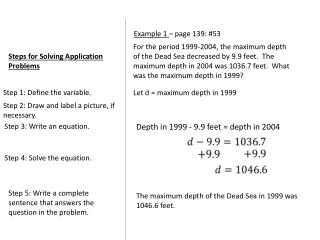DownloadDownload PresentationSteps for Solving Application Problems

# Steps for Solving Application Problems

Download Presentation## Steps for Solving Application Problems

- - - - - - - - - - - - - - - - - - - - - - - - - - - E N D - - - - - - - - - - - - - - - - - - - - - - - - - - -
##### Presentation Transcript

1. Example 1 – page 139: #53 For the period 1999-2004, the maximum depth of the Dead Sea decreased by 9.9 feet. The maximum depth in 2004 was 1036.7 feet. What was the maximum depth in 1999? Steps for Solving Application Problems Step 1: Define the variable. Let d = maximum depth in 1999 Step 2: Draw and label a picture, if necessary. Step 3: Write an equation. Depth in 1999 - 9.9 feet = depth in 2004 Step 4: Solve the equation. Step 5: Write a complete sentence that answers the question in the problem. The maximum depth of the Dead Sea in 1999 was 1046.6 feet.

2. Example 2 – page 139: #55 A rectangular trampoline has a area of 187 square feet. The length of the trampoline is 17 feet. What is its width? Steps for Solving Application Problems Let w = width of the trampoline Step 1: Define the variable. l = 17 ft Step 2: Draw and label a picture, if necessary. w Step 3: Write an equation. Area = length X width Step 4: Solve the equation. Step 5: Write a complete sentence that answers the question in the problem. The width of the trampoline is 11 feet.

3. Practice 1 – page 139: #56 The van used to transport patients to and from a rehabilitation facility is equipped with a wheelchair lift. The maximum lifting capacity for the lift is 300 pounds. The wheel chairs used by the facility weigh 55 pounds each. What is the maximum weight of a wheelchair occupant who can use the lift? Steps for Solving Application Problems Step 1: Define the variable. Step 2: Draw and label a picture, if necessary. Step 3: Write an equation. Step 4: Solve the equation. Step 5: Write a complete sentence that answers the question in the problem.

4. Example 3 – page 145 #37 A dance academy charges \$24 per class and a one-time registration fee of \$15. A student paid a total of \$687 to the academy. Find the number of classes the student took. Steps for Solving Application Problems Step 1: Define the variable. Let n = number of classes the student took Step 2: Draw and label a picture, if necessary. Total paid Number of classes Registration fee rate per class + = x Step 3: Write an equation. Step 4: Solve the equation. Step 5: Write a complete sentence that answers the question in the problem. The student paid for 28 dance classes.

5. Practice 2 – page 145 #38 Tyler paid \$125 to get his car repaired. The total cost for the repairs was the sum of the amount paid for parts and the amount paid for labor. Tyler was charged \$76 for parts and \$32 per hour for labor. Find the amount of time it took to repair his car. Steps for Solving Application Problems Step 1: Define the variable. Step 2: Draw and label a picture, if necessary. Step 3: Write an equation. Step 4: Solve the equation. Step 5: Write a complete sentence that answers the question in the problem.

6. Assignment: Workbook page 31: 21, 22, 23, 24 page 33: 19, 20 Work on notebook paper. Show all steps.